# Central Force Motions (Part - 2)- Classical Mechanics, UGC - NET Physics Physics Notes | EduRev

## Physics : Central Force Motions (Part - 2)- Classical Mechanics, UGC - NET Physics Physics Notes | EduRev

The document Central Force Motions (Part - 2)- Classical Mechanics, UGC - NET Physics Physics Notes | EduRev is a part of the Physics Course Physics for IIT JAM, UGC - NET, CSIR NET.
All you need of Physics at this link: Physics

Centrifugal Energy and the Effective Potential

In equations (6.23) to (6.26) for dr, dt , and θ , respectively, appeared a common term containing different energies (i.e., the total, potential, and rotational energies)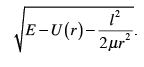(6.41)

The last term is the energy of rotation since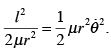(6.42)

It is interesting to note that if we arbitrarily define this quantity as a type of “potential energy” U c , we can derive a conservative force from it. That is, if we set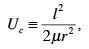(6.43)

then the force associated with it is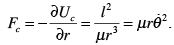(6.44)

The force defined by equation (6.44) is the so-called centrifugal force. It would, therefore, be probably better to call U c is the centrifugal potential energy and to include it with the potential energy U ( r ) to form the effective potential energy V ( r ) defined as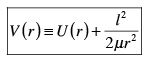(6.45)

If we take for example the case of an inverse-square-law (e.g., gravity or electrostatic), we have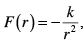(6.46)

and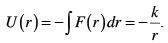(6.47)

The effective potential is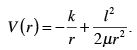(6.48)

It is to be noted that the centrifugal potential “reduces” the effect of the inverse-squarelaw on the particle. This is because the inverse-square-law force is attractive while the centrifugal force is repulsive. This can be seen in Figure 6-2.

It is also possible to guess some characteristics of potential orbits simply by comparing the total energy with the effective potential energy at different values of r . From Figure 6-3 we can see that the motion of the particle is unbounded if the total energy ( E1 ) is greater than the effective potential energy for r ≥ r1 when E1 = V ( r1 ) . This is because the positions for which r < r1 are not allowed since the value under the square root in equation (6.41) becomes negative, which from equation (6.23) would imply an imaginary velocity. For the same reason, the orbit will be bounded with r2 ≤ r ≤ r4 for a total energy E2 , where V ( r2 ) ≤ E2 ≤ V ( r4 ) . The turning points r2 and r4 are called the apsidal distances. Finally, the orbit is circular with r = r3 when the total energy E3 is such that E3 = V ( r3 ) .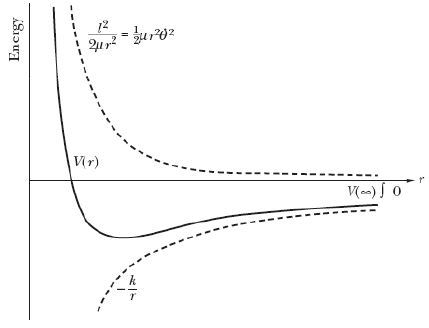Figure 6-2 - Curves for the centrifugal, effective, and gravitational potential energies.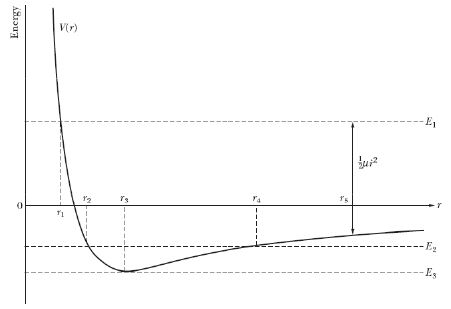Figure 6-3 – Depending on the total energy, different orbits are found.

Planetary Motion – Kepler’s Problem

The equation for a planetary orbit can be calculated from equation (6.26) when the functional form for the gravitational potential is substituted for U ( r )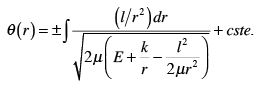(6.49)

This equation can be integrated if we first make the change of variable u = 1 r , the integral then becomes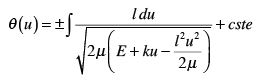(6.50)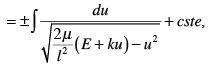the sign in front of the integral has not changed as it is assumed that the limits of the integral were inverted when going from equation (6.49) to (6.50). We further transform equation (6.50) by manipulating the denominator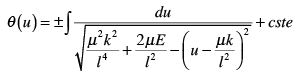(6.51)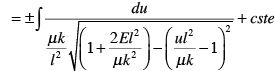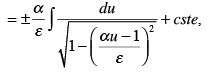with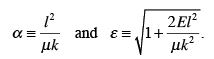(6.52)

Now, to find the solution to the integral of equation (6.51), let’s consider a function f (u )

such that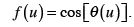(6.53)

Taking a derivative relative to u we get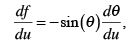(6.54)

or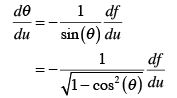(6.55)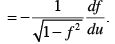Returning to equation (6.51), and identifying f (u ) with the following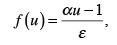(6.56)

we find that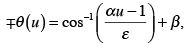(6.57)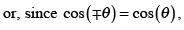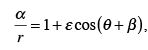(6.58)

where β is some constant. If we choose r to be minimum when θ = 0 , then β = 0 and we finally have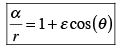(6.59)

with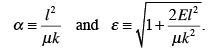(6.60)

Equation (6.59) is that of a conic section with one focus at the origin. The quantities ε and 2" are called the eccentricity and the latus rectum of the orbit, respectively. The minimum value of r (when θ = 0 ) is called the pericenter, and the maximum value for the radius is the apocenter. The turning points are apsides. The corresponding terms for motion about the sun are perihelion and aphelion, and for motion about the earth, perigee and apogee.

As was stated when discussing the results shown in Figure 6-3, the energy of the orbit will determine its shape. For example, we found that the radius of the orbit is constant when E = Vmin . We see from, equation (6.60) that this also implies that the eccentricity is zero (i.e., ε = 0 ). In fact, the value of the eccentricity is used to classify the orbits according to different conic sections (see Figure 6-4):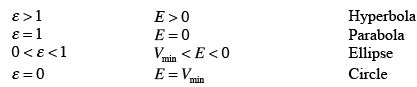For planetary motion, we can determine the length of the major and minor axes (designated by 2a and 2b ) using equations (6.59) and (6.60). For the major axis we have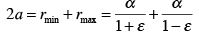(6.61)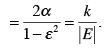For the minor axis, we start by defining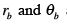as the radius and angle where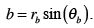(6.62)

Accordingly we have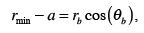(6.63)

which can be written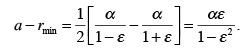(6.64)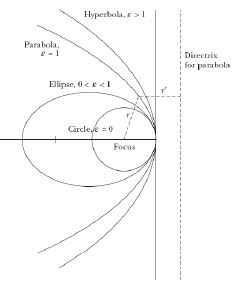Figure 6-4 – The shape of orbits as a function of the eccentricity.

But from equation (6.59) we also have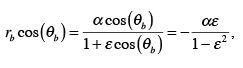(6.65)

or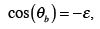(6.66)

and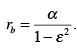(6.67)

Inserting equations (6.66) and (6.67) into equation (6.62) we finally get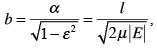(6.68)

or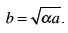(6.69)

The period of the orbit can be evaluated using equation (6.20) for the areal velocity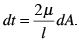(6.70)

Since the entire area enclosed by the ellipse will be swept during the duration of a period ζ , we have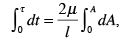(6.71)

or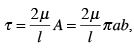(6.72)

where we have the fact that the area of an ellipse is given by πab . Now, substituting the first of equations (6.60) and equation (6.69) in equation (6.72) we get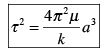(6.73)

In the case of the motion of a solar system planet about the Sun we have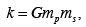(6.74)

where G, m p , and ms are the universal gravitational constant, the mass of the planet, and the mass of the Sun, respectively. We therefore get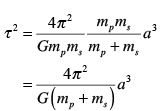(6.75)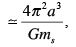since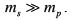. We find that the square of the period is proportional to the semi-major axis to the third, with the same proportionality constant for every planet. This (approximate) result is known as Kepler’s Third Law. We end by summarizing Kepler’s Laws

I. Planets move in elliptical orbits about the Sun with the Sun at one focus.
II. The area per unit time swept out by a radius vector from the Sun to a planet is constant.
III. The square of a planet’s period is proportional to the cube of the major axis of the planet’s orbit.

Offer running on EduRev: Apply code STAYHOME200 to get INR 200 off on our premium plan EduRev Infinity!

159 docs

,

,

,

,

,

,

,

,

,

,

,

,

,

,

,

,

,

,

,

,

,

,

,

,

;